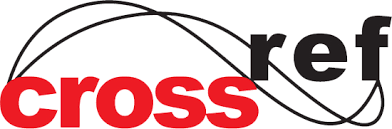# Emphasis on Mathematical Modeling: The Problems of Contour Values in Calculating the Deflection of a Beam

( Vol-5,Issue-12,December 2018 ) OPEN ACCESS
Author(s):

### Edel Alexandre Silva Pontes

Keywords:

Mathematics Teaching and Learning. Contour problems. Deflection and Beams.

Abstract:

Mathematical Modeling has played a fundamental role in the process of teaching and learning mathematics at the various levels of education. The great challenge of today's education is to create means to minimize the distance between the relations of mathematical theory and practical models of everyday life. This work aimed to treat the study of Ordinary Differential Equations through a very usual model of civil construction. Often, students of Mathematics Degree develop skills to understand the abstractions of the vast theory associated with the areas of mathematics, but without being able to exemplify and / or correlate with everyday models.DOI:

10.22161/ijaers.5.12.2

Paper Statistics: